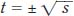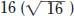NextPrevious

# What are square and square roots?

When you multiply a real or complex number by itself, you are actually squaring that number. Mathematicians express the square of a number using the superscript 2, or, for example, 22. The square of a real number is always positive, whether the number is 22 (= 4) or -22 (= 4; a negative times a negative equals a positive real number).

A square root is a number that when multiplied by itself equals a specific product. For example, if t2 = s, then, in which t is the square root and s is a positive number. For example, the two square roots ofare 4 and -4, as 42 = 16 and -42 = 16.

Close

This is a web preview of the "The Handy Math Answer Book" app. Many features only work on your mobile device. If you like what you see, we hope you will consider buying. Get the App